# The Normal Distribution

### (Chapter 6 in Zar, 2010)

Inferring probabilities from data distributions (that's what we did last week...remember?) can be useful in a descriptive sense, but for inferential statistics we will be making use of theoretical distributions that we can apply to our null expectations. For example, if we were interested in determining whether two sample means represent different statistical populations with different population means, or two samples from a single population (read that again...this is the question that we are asking when we compare means to see if they differ), we would want to define the probability distribution for the difference between 2 sample means drawn from the same population. This is the null expectation, because it is defined by the condition where both sample means estimate the same population mean, rather than each sample mean representing a different statistical population. We use the null expectation because it is an efficient way of making a comparison. There is only one way that 2 sample means can represent a single statistical population, which means that we only have to consider one distribution, that of: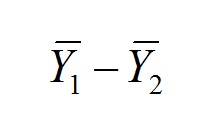Where both sample means were drawn from a single statistical population. From this distribution, we can determine whether a difference that we observe is too improbable (remember that we defined this earlier as a probability less than 0.05) for us to accept the premise that both sample means were, in fact, drawn from the same statistical population.

Considering the probability distributions for the same difference where the two sample means were drawn from statistical populations with two different central tendencies produces an infinite number of possible distributions (one for each amount by which the two population means might differ). Thus, having only a single distribution to deal with (where both sample means estimate the same population mean) makes our analysis (and therefore our lives) much less complicated.

One probability distribution that (under certain specific circumstances that we will concern ourselves with later) does describe the distribution of differences between sample means drawn from a single population is the normal (or Gaussian) distribution. It is important that we become familiar with this distribution and its characteristics, as it plays an integral role in the assumptions of many of the analyses that we will learn. The normal distribution looks like this: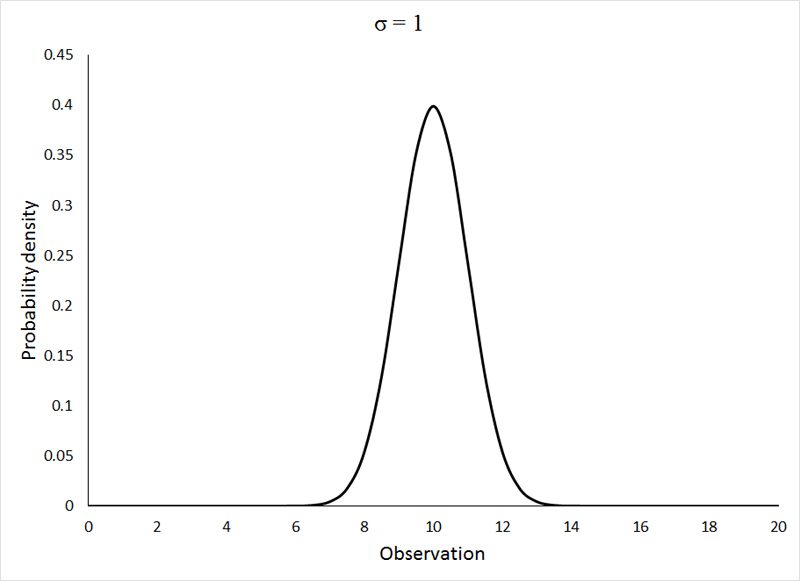All 3 of the above distributions were drawn from a statistical population with μ = 10, and the standard deviation (σ), as indicated on the graphs themselves, varied from 1 to 3. If the change in shape of the distribution with increasing variance surprises you, please go back and review the section on descriptive statistics. If the animation is not working, or if you wish to view the graphs individually, you can view them HERE.

The normal distribution is clearly a symmetrical distribution, but not all symmetrical distributions can be considered to be normal. While all 3 of the above distributions may appear different, they are, in fact, all identical in one regard. The distribution of the observations around the mean is very precisely defined as:

68.27% of the observations lie within 1 standard deviation of the mean ( μ ± σ)

95.45% of the observations lie within 2 standard deviations of the mean ( μ ± 2σ)

99.73% of the observations lie within 3 standard deviations of the mean ( μ ± 3σ)

Or, in a slightly more usable format:

50% of the observations lie within 0.674 standard deviations of the mean ( μ ± 0.674σ)

95% of the observations lie within 1.960 standard deviations of the mean ( μ ± 1.960σ)

99% of the observations lie within 2.576 standard deviations of the mean ( μ ± 2.576σ)

For this reason, the values of the normal distribution (and other probability distibutions that we will employ in our analyses) typically are reported as standardized deviates: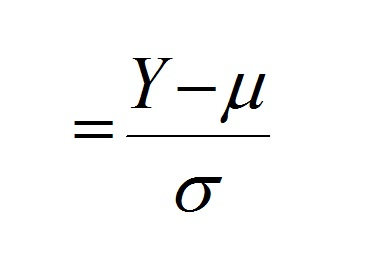Reporting the values as deviates (Y - μ) centers the distribution around zero, and dividing the deviate by the standard deviation (σ) expresses the X-variable (distance from the mean) in units of standard deviation. Applying this calculation to any of the 3 distributions shown above (or any normal distribution for that matter) produces the following distribution:Many observations of biological processes and characteristics tend to follow a normal distribution. One potential reason for this is that these processes and characteristics tend to be influenced by numerous determinants and, if the effects of these determinants are additive, the resulting distribution should approach the parameters of a normal distribution. Let us recall Pascal's triangle and consider multiple draws from binomial probabilities of:

p = q = 0.5

Each draw (remember that k is the number of draws) could represent a different genetic (one of 2 alleles) or environmental (one of 2 conditions) factor that influences a particular character. The probability p reflects the chance that a particular effect adds to that character, such that the value for a character is the sum of all the positive influences on that character. Conversely, q is the probability that the factor does not affect the character. If we assign a value of 1 for each addition to the character, then for a character influenced by only 2 factors, i.e., (p + q) k where k = 2, we would expect the distribution of values for that character to reflect a value of 2 with a probability equal to p2 (0.25 in our case), a value of 1 with a probability equal to 2pq (0.5 in our case), and a value of 0 with a probability q2. This produces a symmetrical, but not normal, distribution.

The more factors influencing the value of the character, i.e., the greater k becomes, the closer the distribution of the values for that character approaches a normal distribution, as is demonstrated below, where the bars represent the distribution of values, and the red line is the expected normal distribution (generated using the NORM.DIST function in Excel) for the same mean and standard deviation: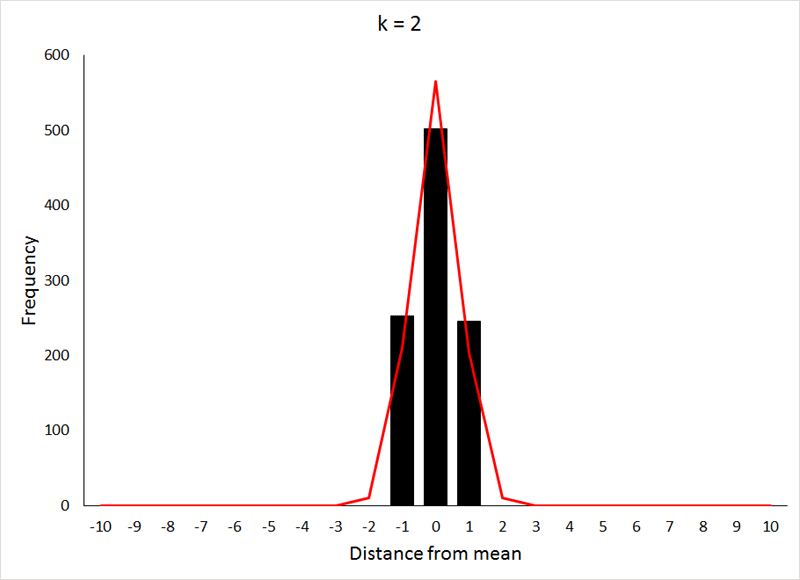The X-axis values in this case are displayed as distances from the mean, because the mean value of the character increases as k increases (the expected mean is pk). The data for the preceding animation were based on 1000 samples from binomial expansions with p = 0.5, and values of k as shown in the graphs. If the above animation isn't working, or if you would like to take a closer look at the graphs, they are shown individually HERE.

This relatively rapid approach to a normal distribution is the result of p being equal to 0.5, which makes the distribution symmetrical at all values of k. For values of p other than 0.5, the approach to a normal distribution occurs much more slowly, as can be seen below (for p = 0.2) by comparing the values for k to those from the previous demonstration:The data for the preceding animation were based on 1000 samples from binomial expansions with p = 0.2, and values of k as shown in the graphs. If the above animation isn't working, or if you would like to take a closer look at the graphs, they are shown individually HERE. The data used in both the preceding animations were generated in R using THIS program.

Question 1: Explain why many biological variables would be expected to exhibit a normal distribution.

It was noted above that the Excel function NORM.DIST was used to generate the red lines indicating the probability densities for the normal distribution given a specifed mean and standard deviation. The syntax for the function is:

=NORM.DIST(x,mean,s,false)

Where x is the value on the X-axis for which you wish to find the probability density. The logical argument at the end should be "false", unless a cumulative probability (as shown by the red line below) is desired:Question 2: What is the relationship between the density function (black line above) and the cumulative density function (red line above)?

If you prefer pencil and paper to Excel functions, the normal probability density function can be calculated as: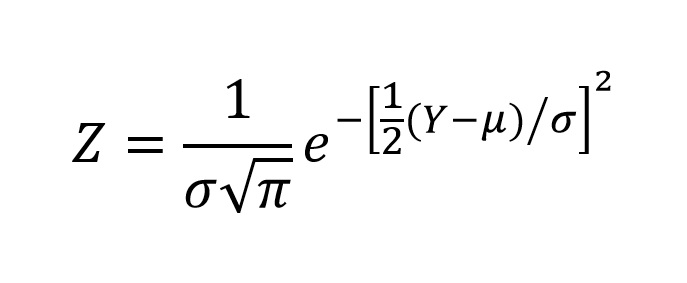While we will make no real use of the normal distribution as a probability distribution for our inferential statistical analyses (which is why I am not putting you through the busy work of generating z-scores, another term for probability densities for the normal distribution), the assumption that our observations are normally distributed will be required for most of our analyses. Although it may seem counterintuitive, we always test our assumptions. One might argue that they no longer should then be considered "assumptions", but that misinterpretation can easily be corrected by realizing that the assumptions are the assumptions of the analysis, and define the conditions under which the analysis will give us a result that can be properly interpreted. That is why we must test our data against those assumptions in order to determine whether the conclusion to which our analysis leads us is an appropriate one.

We have, in a sense, already evaluated several distributions for normality by a visual comparison of the bars to the red lines. Such a comparison, however, is strongly influenced by the size of a graph. As a young and impressionable lad, I was taught that draws from a binomial distribution, as was demonstrated above, would produce a distribution not distinguishable from a normal distribution on a graph printed on 8.5" x 11" paper when k > 25. This is absolutely true, but in the examples I used above, normality was not achieved until k ≈ 200 when p = 0.5, and when k ≈ 600 for p = 0.2. While this specific set of circumstances might not be broadly applicable, it does serve to illustrate the point that one must be cautious with visual comparisons.

The preceding narrative should also have suggested to you that there are other ways to test for normality. One possibility is generating the probability densities and using a Goodness-of-Fit test to compare the observed frequencies to those expected for a normal distribution. We will deal with such approaches later on when we explore "Analysis of Frequencies" in week 13. For now, take comfort in the fact that there is a far better approach.

The Shapiro-Wilk statistic is the most reliable, and most widely applied test for normality. Unfortunately (although you might think it fortunate) it is too cumbersome and computationally intensive for us to do by hand, so when we need to test the assumption of normality, the result of the Shapiro-Wilk test for normality will be provided to you.

One application of the normal distribution (or more correctly, distributions that describe the approach to normality) involves the calculation of confidence intervals...

Send comments, suggestions, and corrections to: Derek Zelmer